Home / Apche Spark

# REPL Environment for Apache Spark Shell

Rating: 5.0Views: 9514
by Ravindra Savaram

## REPL With Apache Spark Shell

In this chapter, we will discuss about one effective feature of Apache Spark, which makes it a convenient tool for both investigative and operational analytics. It is the Read Evaluate Print Loop – REPL environment of Spark Shell, in Scala. We will discuss how it is useful for different analysis tasks with examples.

At MindMajix, we offer resources that help you in learning various IT courses. We avail both written material and demo video tutorials. To gain in-depth knowledge and be on par with practical experience, then explore Apache Spark Certification Training.

REPL: Read Eval Print Loop environment

If you are familiar with any functional programing language like LISP or Haskell, you could have heard above term. It is a programming environment, which accepts inputs  (commands) from a single user in the form of an expression, evaluates the command and prints the result. Command Line Interfaces (CLIs) like MS DOS and scripting languages like Python, BASH shell, etc. are examples of such environments. The term REPL, was originated to refer to the behavior of LISP primitive functions.

To be run in REPL, environment, and the code need not be compiled and executed. User can enter expressions to be evaluated and REPL will display the result after evaluating the expression, as it goes. The ‘Read’ refers to reading the expression as an input and parsing it to an internal data structure and storing it in memory. ‘Eval’ refers to traversing the data structure and evaluating the functions being called. ‘Print’ refers to displaying the results to the user, pretty printing them if needed. It iterates in a ‘Loop’, going back to read state and terminates the loop upon program exit.

REPL environment is quite useful for instant evaluation of expressions and for debugging. Since it does not have to be edited-compiled and run for each modification, REPL functions are faster.

REPL with Apache Spark

As far as data analysis is considered, we perform two types of tasks as investigative and operational. Investigative analysis is done using tools like R or Python, which are suitable for finding answers fast and interactively providing quick insights on the system. Operational analysis refers to the design and implementation of models for large-scale application and are mostly done in high level language like Java or C++.

So it is apparent that while one tool is quite suitable for an ad-hoc analysis, it may not be feasible to scale in certain environments and vice versa. How good it could be if there is one utility supporting both of them? In fact we do and that is Apache Spark. Not only Apache Spark supports investigative analysis in a REPL environment like R or Python, but also enables operational analysis, supporting distributed and scalable solutions for large-scale applications.

One of the eye catching feature of Apache Spark is that it provides an interactive REPL environment in SCALA and also enables to use Java libraries within SCALA. You can also use this environment to learn the Spark API interactively.

In Chapter 1, we got hands on in with Spark interactive shell. Let us see some more examples to witness the use of Spark REPL environment in Scala, both in investigative and operational analysis and its support for debugging. All these examples can be run, after launching the Spark shell with below command in your machine.

spark-shell –master local[*]
Replace * with the number of cores in your machine.

Some of the useful commands in the shell are:

help: For displaying usage of supported functions

history: For displaying names of variables or functions previously used, but you forgot.

paste: Paste data to the shell, copied to clip board

Any application being run on Spark is initiated by SparkContext object, which handles the Spark job execution. This SparkContext object is referred by sc in the Spark REPL environment.

Example Applications

Below is how we can run the word count application in Spark Shell: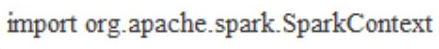val inputFile = sc.textFile(“spark_examples/words.md “)

wordcount.reduceByKey(_ + _).collect().foreach(println)

Next, we will see implementations of some common machine learning algorithms:

K-means algorithm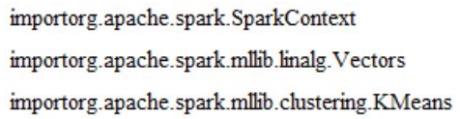// Read data from the file
valdataFile = sc.textFile(“spark_example/kmeans_data_sample.txt”)
val data = dataFile.map(line =>Vectors.dense(line.split(‘ ‘).map(_.toDouble)))
// Kmeans clustering where k = 4
valnumberOfClusters = 4
val count = 20
val clusters = KMeans.train(data, numberOfClusters, count)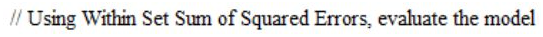valWISetSumofSquaredError = clusters.computeCost(data)
println(“Within Set Sum of Squared Errors for 4 means = ” +
WISetSumofSquaredError)

Linear Regression Algorithm

Implementation of linear regression algorithm in Spark REPL environment is as below: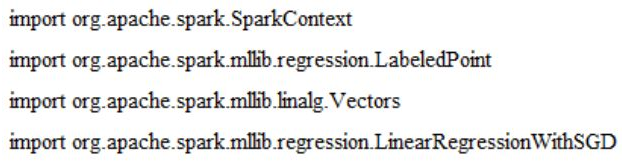// Read data from the file
val dataFile = sc.textFile(“spark_example/linear_regression_data_sample”)
val data = dataFile.map { lineRead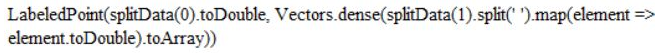}

// Model building
val count = 40
val model = LinearRegressionWithSGD.train(data, count)
// While using the test data – one can evaluate model to find out test error.
val predictedVals = data.map { modelElement =>
val predictedValue = model.predict(modelElement.features)
(modelElement.label, predictedValue)
}
val MeanSquareError = predictedVals.map{ case(w, pow) => math.pow((w – pow),
2)}.reduce(_ + _)/predictedVals.count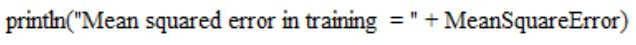Support Vector Machines (SVM) Algorithm

Learn Apache Spark Tutorial

Implementation of SVM algorithm in Spark REPL environment is as below.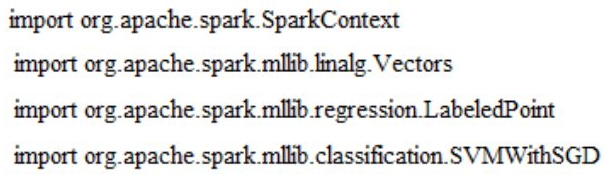val dataFile = sc.textFile(“spark_example/svm_data_sample”)
val data = dataFile.map { lineRead =>
LabeledPoint(splitData(0).toDouble, Vectors.dense(splitData(1).split(‘ ‘).map(element
=> element.toDouble).toArray))
}

val count = 40

// While using the test data – one can evaluate model to find out test error.
val predictedVals = data.map { modelElement =>
val predictedValue = model.predict(modelElement.features)
(modelElement.label, predictedValue)
}
val trainingError = predictedVals.filter(r => r._1 != r._2).count.toDouble / data.count
println(“Training Error = ” + trainingError)

Decision Tree Algorithm

Below is the implementation of decision tree for prediction and calculating the error in training model.

val dataFile = sc.textFile(“spark_example/decision_tree_regression_sample.csv”)
val data = dataFile.map { line =>
val splitData = line.split(‘,’).map(_.toDouble)
LabeledPoint(splitData(0), Vectors.dense(splitData.tail))
}

val maximumTreeDepth = 8
val model = DecisionTree.train(data, Regression, Variance, maximumTreeDepth)

// While using the test data – one can evaluate model to find out test error.
val predictedValues = data.map { modelElement =>
val predictedVal = model.predict(modelElement.features)
(modelElement.label, predictedVal)
}
val MeanSquareError = predictedValues.map{ case(w, pow) => math.pow((w – pow),
2)}.mean()

Naïve Bayes Method

Naïve Bayes method, for machine learning can be implemented in Spark shell as below, using the supported APIs.

valdataFile = sc.textFile(“spark_example/naive_bayes_data_sample”)
val data = dataFile.map { lineRead =>
LabeledPoint(splitData(0).toDouble, Vectors.dense(splitData(1).split(‘
‘).map(_.toDouble)))
}

valdataSplits = data.randomSplit(Array(0.7, 0.3), seed = 11L)
valtrainingData = dataSplits(0)
valtestData = dataSplits(1)

valnaiveBayesModel = NaiveBayes.train(trainingData, lambda = 1.0)
val prediction = naiveBayesModel.predict(testData.map(_.features))
vallabelledPredictEl = prediction.zip(testData.map(_.label))
//Calculate and display accuracy of the model
val precision = 1.0 * labelledPredictEl.filter(x => x._1 == x._2).count() /
testData.count()

println(“Precision of the model built = ” + precision)

Using Third Party Libraries in Spark Shell

Third party libraries can be conveniently used within Spark shell. To do this, we need to have the required jar file added to the classpath. The classpath configuration can be done when invoking the spark shell with the option “–driver-class-path” as below./bin/spark-shell –other_options_as_key_value –driver-class-path path_to_the_library

As we saw above, we can perform many data analytics, without having compiled code and use of build tool like maven or sbt. So how can we decide when to use which?

Most of the time, Spark REPL would be sufficient to run your entire application from the beginning. It provides faster execution, quick response and enables to prototype the application quickly. Still, as the application grows with size and complexity and the sequence of code becomes largest, the execution time may increase. Also if you are working with a large amount of data, this may also lead to program fault, wiping out all the variables and functions being used in the current shell, which will lead to cumbersome rework. Therefore, as you forward with the application, it is better to make a hybrid use of both.

At the initial stages with less amount of complex code use SPARK REPL environment for quick analysis and debugging, and as the application expands in data size and complexity, move the implementation to a compiled library and use it in the shell. We have seen above, how we can import compiled libraries to Spark Shell. With this approach, given that compiled library will not need frequent editing and recompiling, running the application in Spark REPL environment will produce faster results.